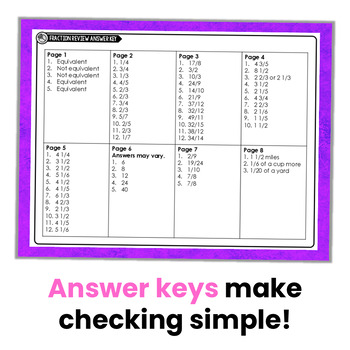5th - 6th
Subjects
Standards
Resource Type
Formats Included
• PDF
Pages
30 pages

#### Also included in

1. Math review can be made just a bit more interesting just by changing up the format. This resource is a bundle of my math review booklets for 4th and 5th grade.Click here to see my review booklets for reading and language.About the 4th and 5th Grade Math Review BookletsI created these 4th and 5th gra
\$10.00
\$19.00
Save \$9.00

### Description

I created this resource to have a ready made resource for re-teaching and reviewing 5th grade fraction skills. My students respond really well to the novelty of printable mini-booklets. It still gives them that pencil-to-paper practice, but in a less threatening format.

To save copies, there are two students page on one page.

This resource does not include steps or instructions for the skills. It’s practice only.

This resource includes a practice page for these 5th grade fraction skills:

Equivalent Fractions

Simplifying Fractions

Mixed Numbers to Improper Fractions

Improper Fractions to Mixed Numbers

Rewriting Mixed Numbers with Improper Fractions

Finding Common Denominators

Adding and Subtracting Fractions with Unlike Denominators

Adding and Subtracting Fractions Word Problems

Subtracting Mixed Numbers {Borrow from the Whole}

Add and Subtract Mixed Numbers Word Problems

Multiplying Fractions by Whole Numbers

Multiplying Fractions by Fractions with Models

Multiplying Fractions

Multiplying Fractions Word Problems

Multiplying Mixed Numbers

Multiplying Mixed Numbers Word Problems

Dividing with Unit Fractions

Dividing with Unit Fractions Word Problems

All Operations Fractions Word Problems

Using the 5th Grade Fractions Booklet

The possibilities for use of this resources are numerous. Here are just a few ideas:

Mini Check Ins or Assessments

Test Prep

Re-teaching

Independent Practice

Centers

RTI

Homework

Morning Work

You may also like:

Multiplying and Dividing Fractions Resources

Fraction Practice Pages

Fraction Spinner Math Centers

Adding and Subtracting Mixed Numbers Resources

Subtracting Mixed Numbers with Regrouping Resources

Fraction Math Mat Centers

Total Pages
30 pages
Included
Teaching Duration
N/A
Report this Resource to TpT
Reported resources will be reviewed by our team. Report this resource to let us know if this resource violates TpT’s content guidelines.

### Standards

to see state-specific standards (only available in the US).
Add and subtract fractions with unlike denominators (including mixed numbers) by replacing given fractions with equivalent fractions in such a way as to produce an equivalent sum or difference of fractions with like denominators. For example, 2/3 + 5/4 = 8/12 + 15/12 = 23/12. (In general, 𝘢/𝘣 + 𝘤/𝘥 = (𝘢𝘥 + 𝘣𝘤)/𝘣𝘥.)
Solve word problems involving addition and subtraction of fractions referring to the same whole, including cases of unlike denominators, e.g., by using visual fraction models or equations to represent the problem. Use benchmark fractions and number sense of fractions to estimate mentally and assess the reasonableness of answers. For example, recognize an incorrect result 2/5 + 1/2 = 3/7, by observing that 3/7 < 1/2.
Apply and extend previous understandings of multiplication to multiply a fraction or whole number by a fraction.
Solve real world problems involving multiplication of fractions and mixed numbers, e.g., by using visual fraction models or equations to represent the problem.
Apply and extend previous understandings of division to divide unit fractions by whole numbers and whole numbers by unit fractions.